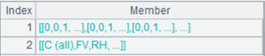# cs.()

cs.(x)

cs.()     返回cs本身

 cs 游标 x 计算表达式，一般为字段名，或者由字段名组成的合法表达式，可使用~引用当前记录。

 A 1 =connect("demo").cursor("select * from EMPLOYEE where EID<10") 2 =A1.(age(BIRTHDAY)) 通过A1中的生日字段BIRTHDAY计算年龄 3 =A2.fetch()4 =connect("demo").cursor("select * from SCORES where SCORE<60") 5 =A4.(SCORE) 6 =A5.(~*~) 用~引用序列当前成员 7 =A6.fetch()8 =demo.cursor("select * from DEPARTMENT") 9 =A8.() 返回A8 10 =A9.fetch()